2 Gauss Law and Applications Coulombs Law force

• Slides: 18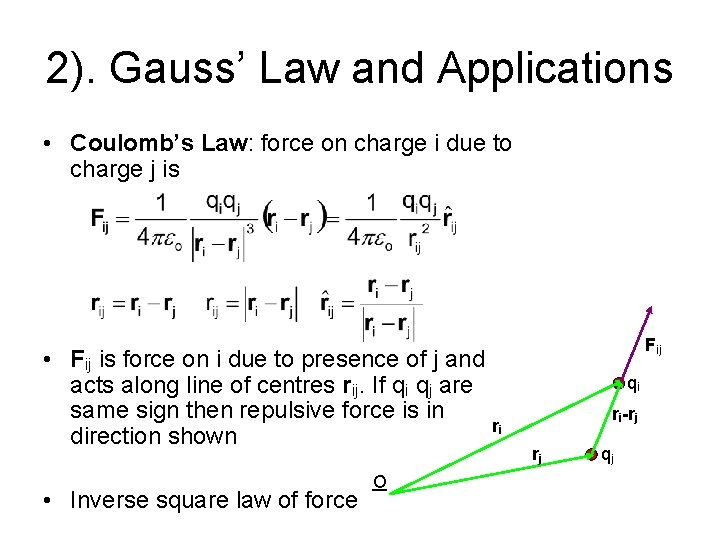2). Gauss’ Law and Applications • Coulomb’s Law: force on charge i due to charge j is • Fij is force on i due to presence of j and acts along line of centres rij. If qi qj are same sign then repulsive force is in ri direction shown • Inverse square law of force O Fij qi ri-rj rj qj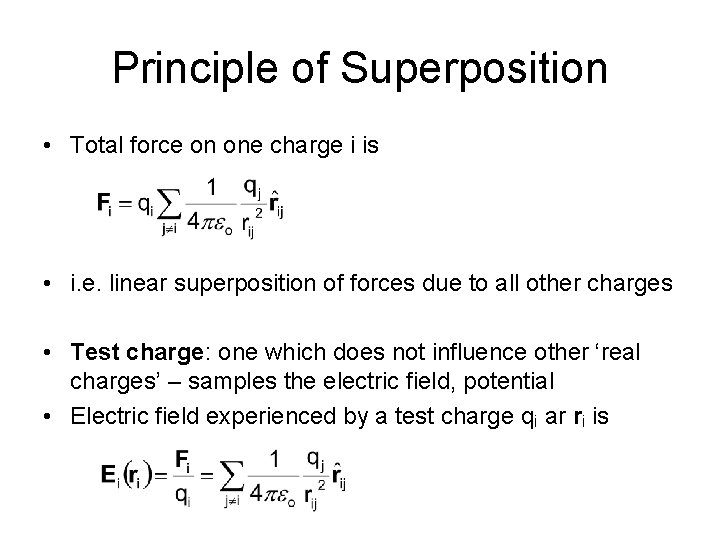Principle of Superposition • Total force on one charge i is • i. e. linear superposition of forces due to all other charges • Test charge: one which does not influence other ‘real charges’ – samples the electric field, potential • Electric field experienced by a test charge qi ar ri is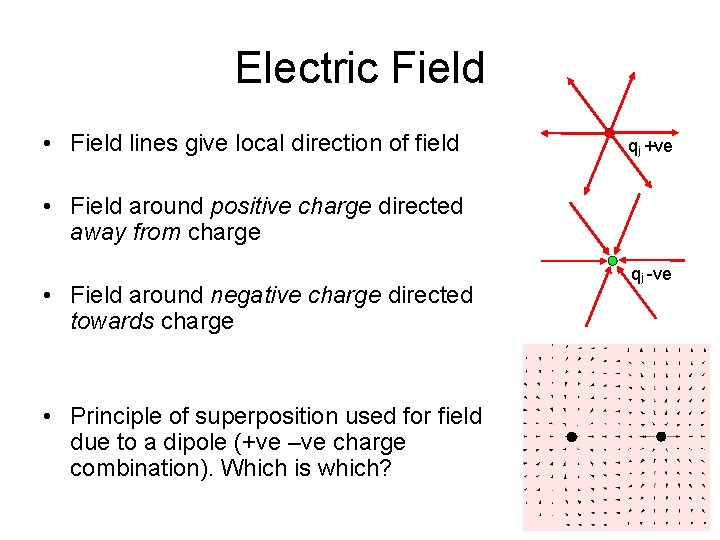Electric Field • Field lines give local direction of field qj +ve • Field around positive charge directed away from charge • Field around negative charge directed towards charge • Principle of superposition used for field due to a dipole (+ve –ve charge combination). Which is which? qj -veFlux of a Vector Field • Normal component of vector field transports fluid across element of surface area • Define surface area element as d. S = da 1 x da 2 • Magnitude of normal component of vector field V is V. d. S = |V||d. S| cos(Y) da 2 • For current density j flux through surface S is Cm 2 s-1 d. S da 1 Y d. S` d. S = da 1 x da 2 |d. S| = |da 1| |da 2|sin(p/2)Flux of Electric Field • Electric field is vector field (c. f. fluid velocity x density) • Element of flux of electric field over closed surface E. d. S n da 2 q da 1 f Gauss’ Law Integral FormIntegral form of Gauss’ Law • Factors of r 2 (area element) and 1/r 2 (inverse square law) cancel in element of flux E. d. S • E. d. S depends only on solid angle d. W n da 2 da 1 q Point charges: qi enclosed by S q 1 f q 2 Charge distribution r(r) enclosed by SDifferential form of Gauss’ Law • Integral form • Divergence theorem applied to field V, volume v bounded by surface S V. n d. S . V dv • Divergence theorem applied to electric field E Differential form of Gauss’ Law (Poisson’s Equation)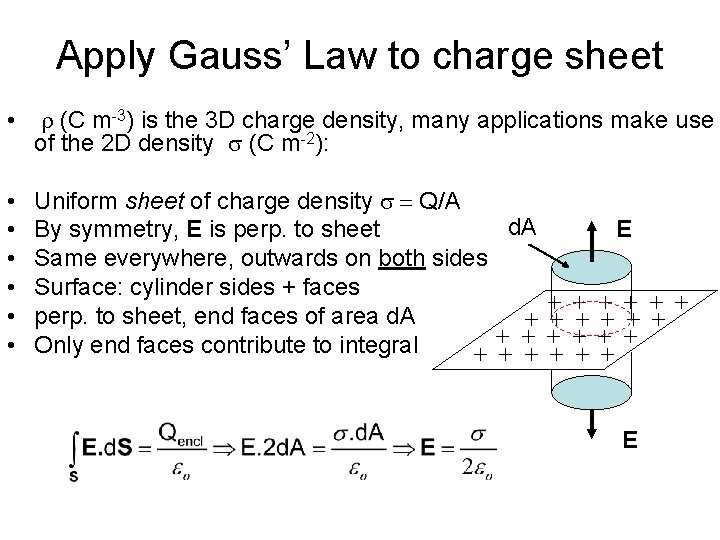Apply Gauss’ Law to charge sheet • r (C m-3) is the 3 D charge density, many applications make use of the 2 D density (C m-2): • • • Uniform sheet of charge density = Q/A d. A E By symmetry, E is perp. to sheet Same everywhere, outwards on both sides Surface: cylinder sides + faces + + + perp. to sheet, end faces of area d. A + + + Only end faces contribute to integral + + + EApply Gauss’ Law to charged plate ’ = Q/2 A surface charge density Cm-2 (c. f. Q/A for sheet) E 2 d. A = ’ d. A/ o E = ’/2 o (outside left surface shown) E = 0 (inside metal plate) why? ? E • Outside E = ’/2 o + ’/2 o = ’/ o = /2 o • Inside fields from opposite faces cancel + + + + d. A + + + + + + • • •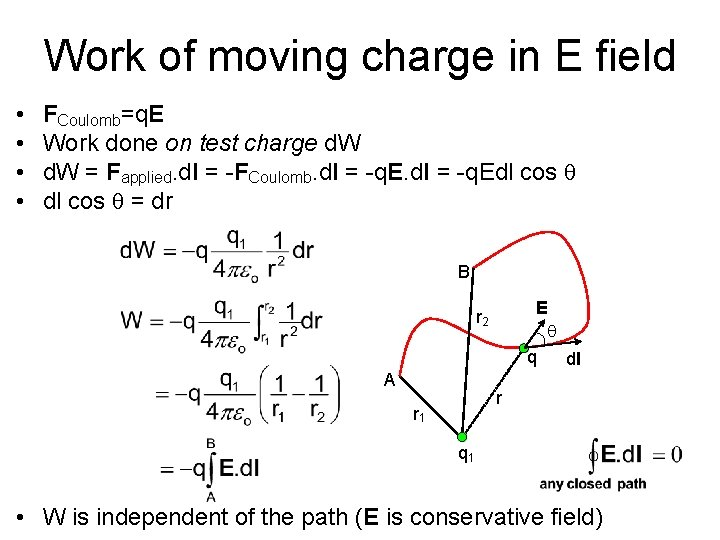Work of moving charge in E field • • FCoulomb=q. E Work done on test charge d. W = Fapplied. dl = -FCoulomb. dl = -q. Edl cos q = dr B E q q dl r 2 A r r 1 q 1 • W is independent of the path (E is conservative field)Potential energy function • Path independence of W leads to potential and potential energy functions • Introduce electrostatic potential • Work done on going from A to B = electrostatic potential energy difference • Zero of potential energy is arbitrary – choose f(r→∞) as zero of energyElectrostatic potential • Work done on test charge moving from A to B when charge q 1 is at the origin • Change in potential due to charge q 1 a distance of r. B from B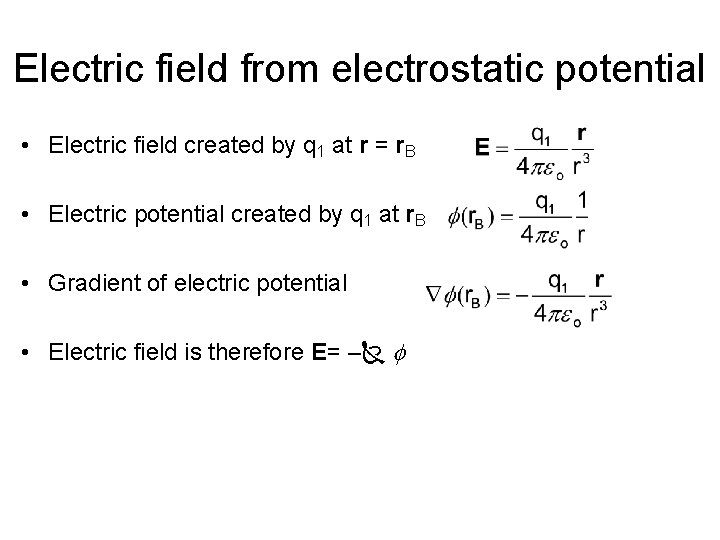Electric field from electrostatic potential • Electric field created by q 1 at r = r. B • Electric potential created by q 1 at r. B • Gradient of electric potential • Electric field is therefore E= – f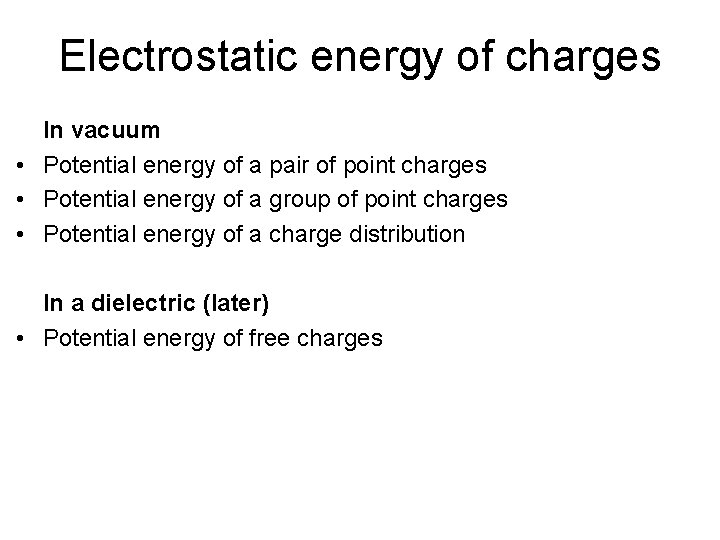Electrostatic energy of charges In vacuum • Potential energy of a pair of point charges • Potential energy of a group of point charges • Potential energy of a charge distribution In a dielectric (later) • Potential energy of free charges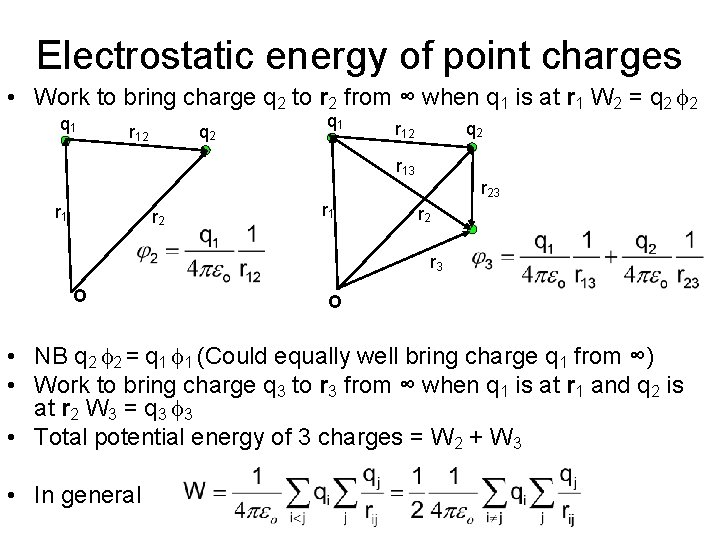Electrostatic energy of point charges • Work to bring charge q 2 to r 2 from ∞ when q 1 is at r 1 W 2 = q 2 f 2 q 1 q 2 r 12 r 13 r 1 r 23 r 2 r 3 O O • NB q 2 f 2 = q 1 f 1 (Could equally well bring charge q 1 from ∞) • Work to bring charge q 3 to r 3 from ∞ when q 1 is at r 1 and q 2 is at r 2 W 3 = q 3 f 3 • Total potential energy of 3 charges = W 2 + W 3 • In general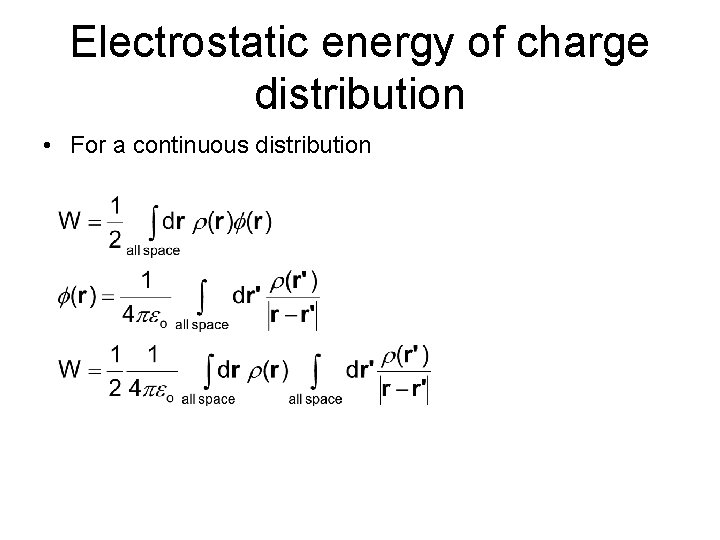Electrostatic energy of charge distribution • For a continuous distributionEnergy in vacuum in terms of E • • Gauss’ law relates r to electric field and potential Replace r in energy expression using Gauss’ law • Expand integrand using identity: . F = . F + F. Exercise: write = f and F = f to show:Energy in vacuum in terms of E For pair of point charges, contribution of surface term 1/r -1/r 2 d. A r 2 overall -1/r Let r → ∞ and only the volume term is non-zero Energy density